+

# Introduction to Slope

##### Rating:
(3)
• (1)
• (1)
• (0)
• (0)
• (1)
Author: Sophia Tutorial
##### Description:

Identify the slope of a line perpendicular to a given line.

(more)
Tutorial
what's covered
1. The Slope of a Line
2. Slopes of Vertical and Horizontal Lines
3. Slopes of Parallel and Perpendicular Lines

# 1. The Slope of a Line

As we graph lines, we will want to be able to identify different properties of the lines we graph. One of the most important properties of a line is its slope. Slope is a measure of steepness. A line with a large slope, such as 25, is very steep. A line with a small slope, such as 0.10 is very flat. We will also use slope to describe the direction of the line. A line that goes up from left to right will have a positive slope and a line that goes down from left to right will have a negative slope.

term to know
Slope
the steepness of a line; found by dividing the change in y-coordinates by the change in x-coordinates from any two points on a line.

As we measure steepness, we are interested in how fast the line rises compared to how far the line runs. For this reason we will describe slope as the fraction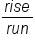. Rise would be a vertical change, or a change in the y-values. Run would be a horizontal change, or a change in the x-values. So another way to describe slope would be the fraction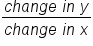. It turns out that if we have a graph, we can draw vertical and horizontal lines from one point to another to make what is called a slope triangle. The sides of the slope triangle give us our slope. The following examples show graphs that we find the slope of using this idea.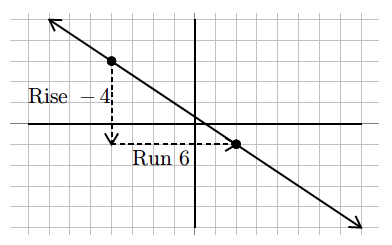To find the slope of this line, we will consider the rise, or vertical change and the run or horizontal change. Drawing these lines in makes a slope triangle that we can use to count from one point to the next the graph goes down 4, right 6. This is rise − 4, run 6. As a fraction it would be,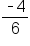. Reduce the fraction to get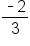.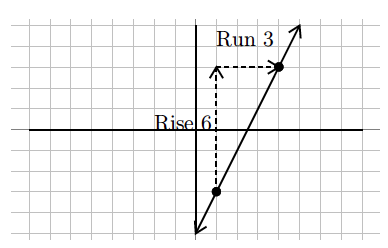To find the slope of this line, the rise is up 6, the run is right 3. Our slope is then written as a fraction, rise over run or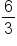. This fraction reduces to 2, so the slope is 2.

We can find the slope of a line through two points without seeing the points on a graph. We can do this using a slope formula. If the rise is the change in y values, we can calculate this by subtracting the y values of a point. Similarly, if run is a change in the x values, we can calculate this by subtracting the x values of a point. In this way we get the following equation for slope.

 The slope of a line through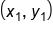and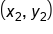is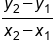When mathematicians began working with slope, it was called the modular slope. For this reason we often represent the slope with the variable m. Now we have the following for slope.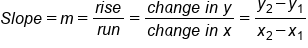formula
Slope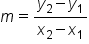# 2. Slopes of Vertical and Horizontal Lines

There are two special lines that have unique slopes that we need to be aware of. The line below is a horizontal line.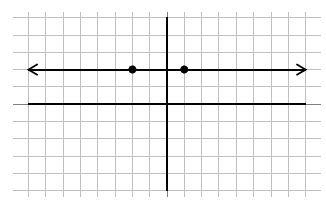For this line, the slope is not steep at all, in fact it is flat. Therefore it has a zero slope. All horizontal lines have a zero slope.

This next line is a vertical line.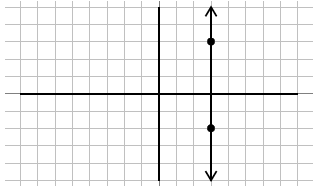For this line, the slope can’t get any steeper. It is so steep that there is no number large enough to express how steep it is so this is an undefined slope. All vertical lines have an undefined slope.

As you can see there is a big difference between having a zero slope and having no slope or undefined slope. Remember, slope is a measure of steepness.

# 3. Slopes of Parallel and Perpendicular Lines

There is an interesting connection between the slope of lines that are parallel and the slope of lines that are perpendicular (meet at a right angle). This is shown in the following example.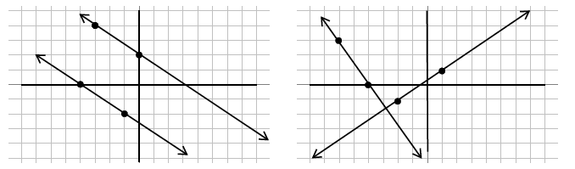As the above graphs illustrate, parallel lines have the same slope and perpendicular lines have opposite (one positive, one negative) reciprocal (flipped fraction) slopes. We can use these properties to make conclusions about parallel and perpendicular lines.

Let's take a further look at the example above with perpendicular lines.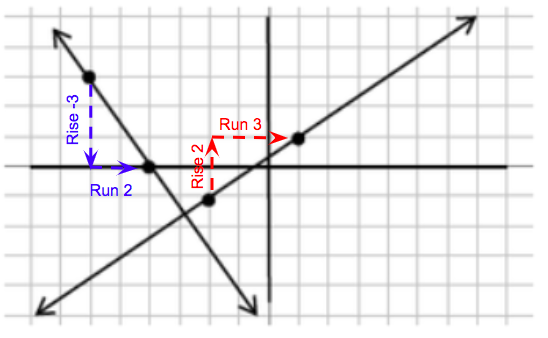One line has a rise of -3 and a run of 2, so the slope is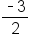. The other line that is perpendicular has a rise of 2 and a run of 3, so the slope of this line is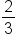. These two slopes are opposite reciprocals. They are opposite meaning one slope is positive while the other is negative. The slopes are also reciprocals meaning their fractions are flipped.

terms to know
Parallel lines
lines that never intersect; they have identical slopes
Perpendicular lines
lines that intersect at a right angle; they have opposite reciprocal slopes

summary
The slope of a line is a measure of how steep it is. When reading a graph from left to right, lines with a negative slope head towards negative infinity on the y-axis and lines with a positive slope head towards positive infinity on the y-axis. The slopes of vertical and horizontal lines are unique. Horizontal lines have a slope of zero and vertical lines have a slope that is undefined. The slopes of parallel and perpendicular lines are special too. Parallel lines have the same slope and perpendicular lines have slopes that are opposite reciprocals of each other.

Terms to Know
Parallel Lines

lines that never intersect; they have identical slopes

Perpendicular Lines

lines that intersect at a right angle; they have opposite reciprocal slopes

Slope

the steepness of a line; found by dividing the change in y-coordinates by the change in x-coordinates from any two points on a line

Formulas to Know
SlopeRating Header